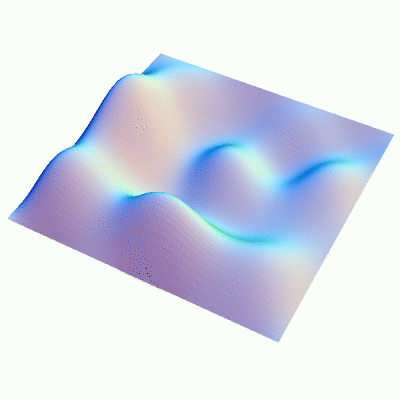Fall 2003

# Mathematics Math21b Fall 2003

## Linear Algebra and Differential Equations

Office: SciCtr 434
Email: knill@math.harvard.edu# 2D linear wave equation

The wave equation on a plate in two dimensions

 ``` ftt(t,x,y) = fxx(t,x,y) + fyy(t,x,y) ```
can be solved similar as the one dimensional case. Take the basis fn,m = sin(n x) sin(m y) for functions on the square [0,pi] x [0,pi] which vanish at the boundary. The wave equation can be written as ftt = A f. Because
 ```A fn,m=(-n2-m2) fn,m ```
we have
 ```fn,m(t)=cos(k t)fn,m(0)+sin(k t)f'n,m(0)/k ```
with k=|(n,m)|. The general solution is a superposition of such waves. The wave is periodic in time only if all pairs (n,m) with nonzero Fourier coefficients are Pythagorean pairs: n2 + m2 = k2.Back to the main page